# Graphing linear equations

In this mini-lesson, we will explore solving a system of graphing linear equations using different methods, linear equations in two variables, linear equations in one variable, solved examples, and pair of linear equations.

For example, $$2x-5y+21=0$$ is a linear equation.

But how do you represent linear equations on a graph?

To plot a graph of linear equations in two variables, we have different methods.

Let us learn in detail about them in the following lesson.

## Lesson Plan

 1 What Do You Mean by Graphing Linear Equations? 2 Important Notes on Graphing Linear Equations 3 Solved Examples 4 Interactive Questions 5 Challenging Questions

## What Do You Mean by Graphing Linear Equations?

Linear equations are algebraic equations in which each term has a real constant and the equation contains 2 variables of highest power 1.
We represent the linear equation in $$y=mx+b$$ form, also known as the y-intercept form.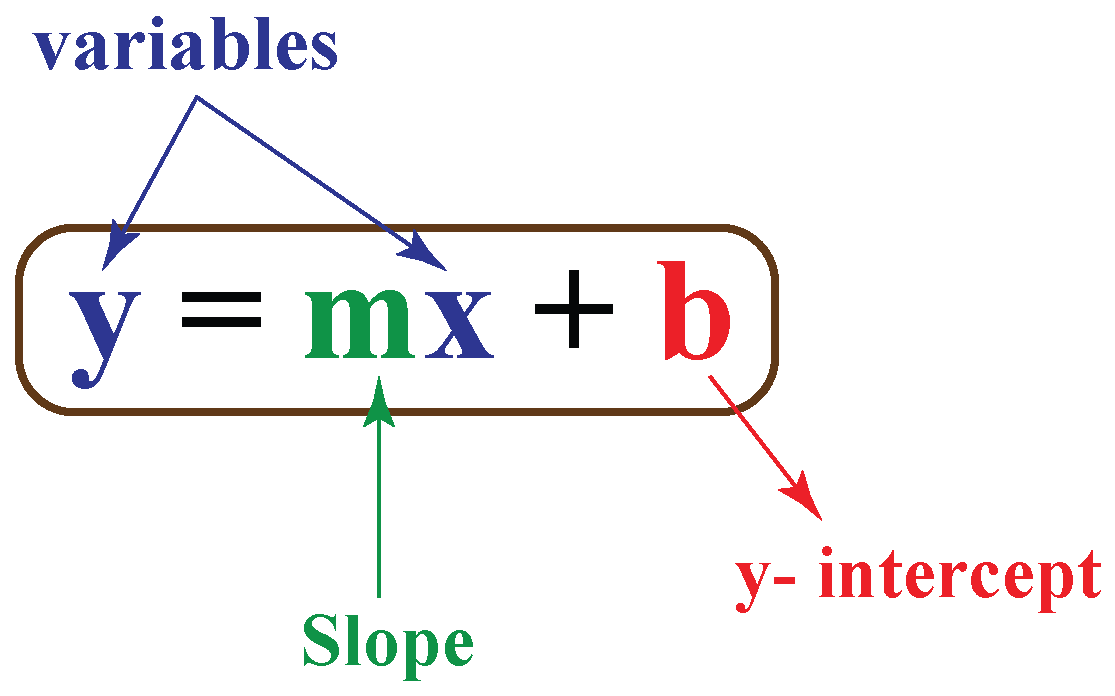The representation of a linear equation on a graph is called a graphing linear equations in two variables.

Also read:

What are Linear Equations in One Variable?-Olympiad

Let us see an example of graphing a linear equation:

We have to represent the equation $$x+2y=7$$  in graph.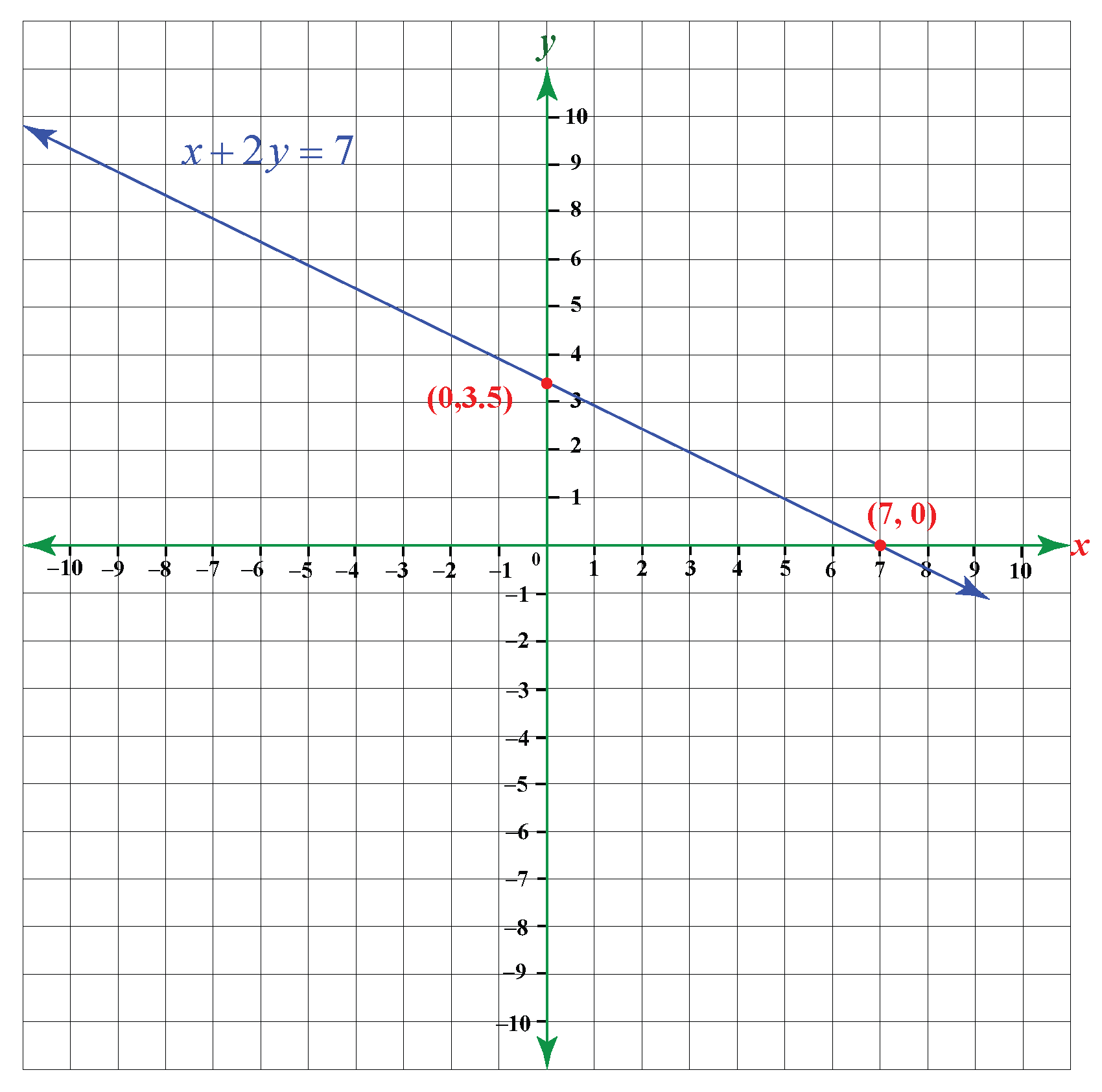Here, the equation $$x+2y=7$$ makes a straight line on the graph.

Similarly, all linear equations create a straight line on the graph.

Note: X-intercept is the point where any line crosses the x-axis on the graph.

Y-intercept is the point where any line crosses the y-axis on the graph.

## How Do You Represent Linear Equation on Graph?

• Make sure the linear equation is in y-intercept form, which is $$y = mx + b$$.
• Apply the trial and error method and find the value of (x, y) up to three pairs, which satisfy the linear equation.
• Find the x-intercept and y-intercept of the equation.
For y-intercept, substitute the value of x = 0 in the equation. This results in x = a.
For x-intercept, substitute the value of y = 0 in the equation. This results in y = c.
• Thus, the points are (a, 0) and (0, c). Make a tabular form and put the value of x and y respectively.
• Plot all the points on the graph paper.
• Join all the points which are marked on the graph paper and get a straight line that represents the given linear equation graphically.

Let's take an example:

Example

Draw a graph of the linear equation $$x+2y=7$$.

Solution

We have to convert the given linear equation $$x+2y=7$$ in the form of y = mx + b.
On converting, we get: $$x=7-2y$$
We need to find the x and y-intercept respectively.
For that, put y=0 in the equation.
$$x=7-2(0)$$
$$x=7$$

Put x=0 in the equation.
$$2y=7-(0)$$
$$y=\dfrac72 = 3.5$$
Now, we will apply the trial and error method and find 3 pairs of values of (x, y) which satisfy the given linear equation $$x=7-2y$$

See the values of x and y in the following table.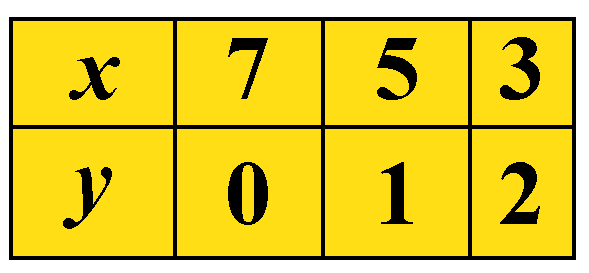Plot the points (7,0), (5,1), and (3,2) on the graph.

Join all the points which are marked on the graph paper and get a straight line that represents the given linear equation graphically.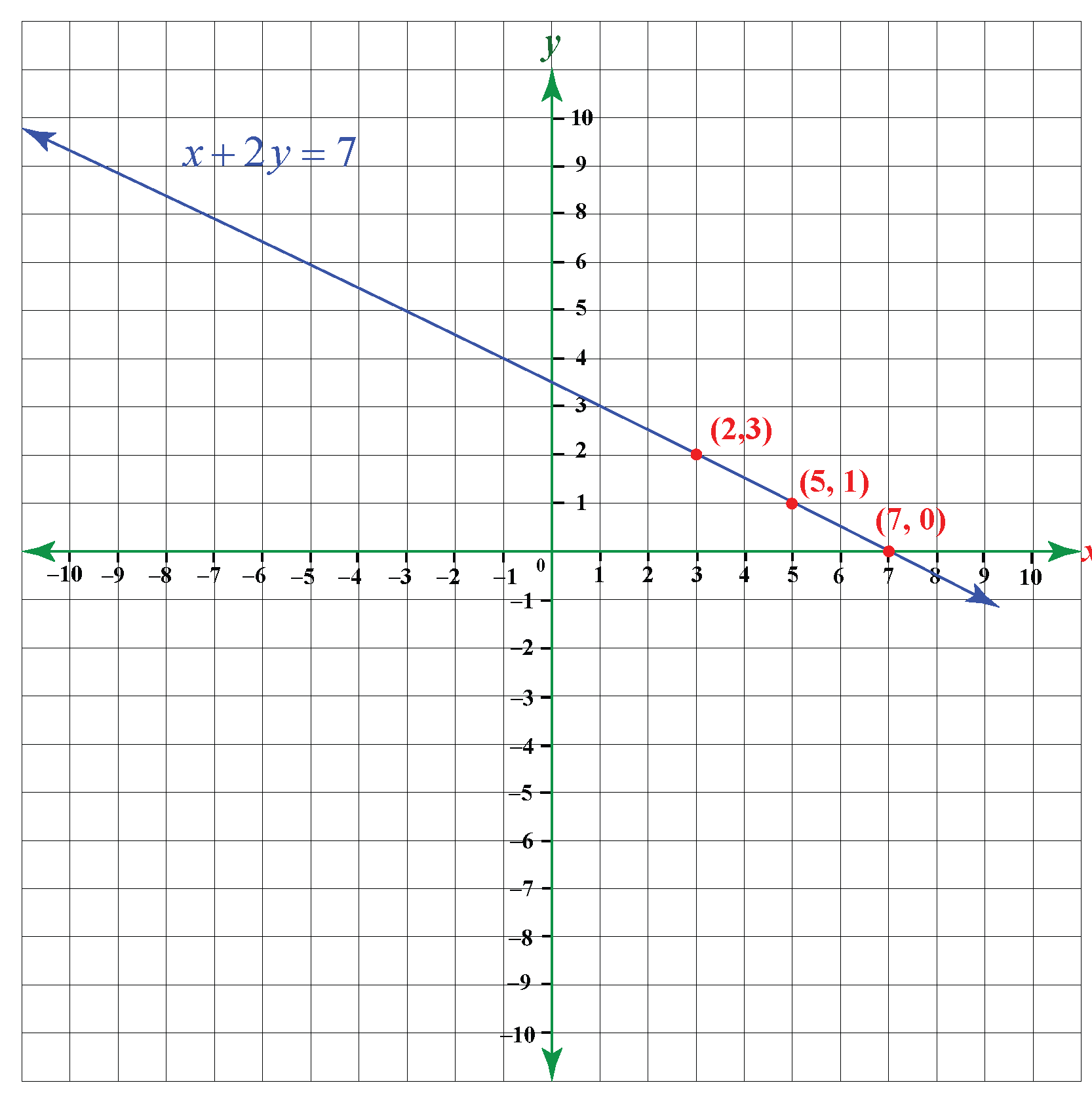Important Notes
• Linear equation in two variables has infinitely many solutions.
• A graph of linear equation is always a straight line.
• The equation y = mx is always passing through the origin (0, 0).

## Solved Examples

 Example 1

Isabella has the linear equation x - 2y = 2. Help her to draw the linear equation on the graph.

Solution

The given linear equation is $$x-2y=2$$. Convert the equation in the form of y = mx + b.
$y=\dfrac x2 - 1$

We need to find the x and y-intercept respectively.

For that, put y=0 in the equation.
$x=2(0)+2$
$x=2$

Put x=0 in the equation.
$2y=(0) - 2$
$$y=1$$

Now, we will apply the trial and error method, and find 3 pairs of values of (x, y) which satisfy the given linear equation $$y=\dfrac x2 - 1$$.
See the values of x and y in the following table.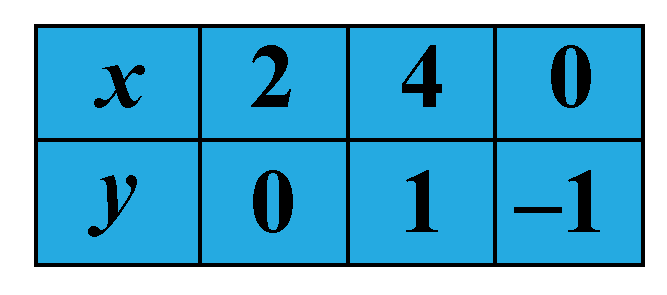Plot the points (2,0),(4,1),(0,-1) on the graph.

Join all the points which are marked on the graph paper and get a straight line that represents the given linear equation graphically.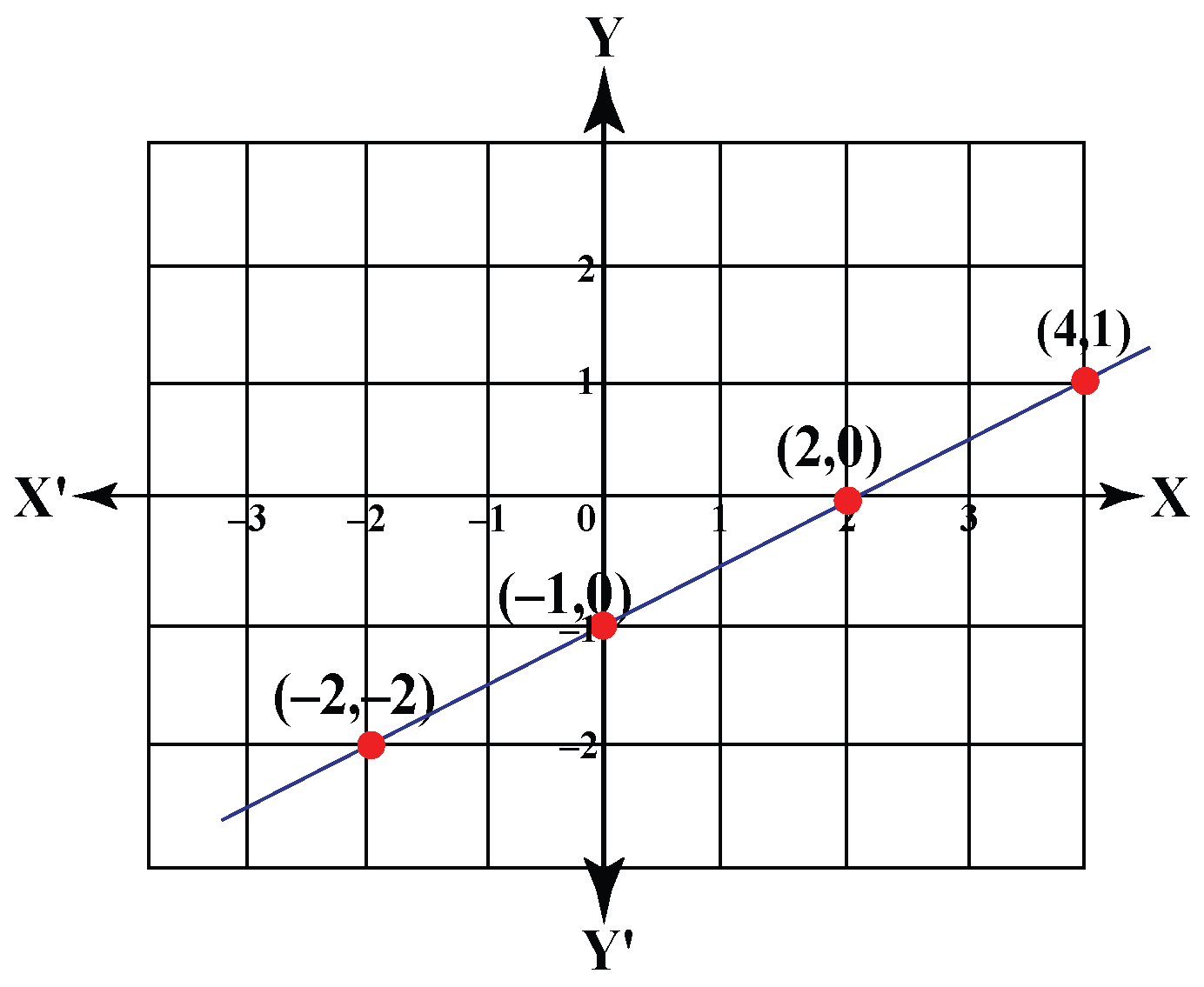Example 2

William wants to plot the graph of $$- \frac{1}{2}x + \frac{1}{3}y = 1$$. Help him to plot the graph for linear equation.

Solution

We determine any two specific points for the solution of the equation:

 $x =$ $$2$$ $$0$$ $y =$ $$6$$ $$3$$ Solution: $\left( {2,6}\right)$ $\left( {0,3}\right)$

The graph of the equation is plotted below: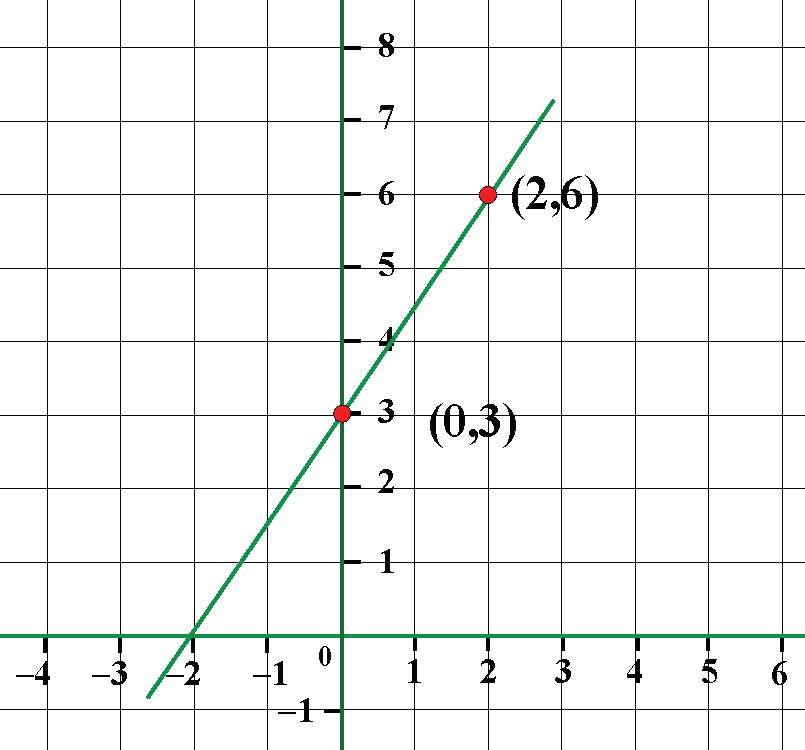Challenging Questions
• The sum of the digits of a two-digit number is 8. When the digits are reversed, the number is increased by 18. Find the number.
• Jake's piggy bank has 11 coins (only quarters or dimes) that have a value of \$1.85. How many dimes and quarters does the piggy bank have?

## Interactive Questions

Here are a few activities for you to practice.

Select/type your answer and click the "Check Answer" button to see the result.

## Let's Summarize

The mini-lesson targeted the fascinating concept of graphing linear equations. The math journey around graphing linear equations starts with what a student already knows, and goes on to creatively crafting a fresh concept in the young minds. Done in a way that is not only relatable and easy to grasp, but will also stay with them forever. Here lies the magic with Cuemath.

At Cuemath, our team of math experts is dedicated to making learning fun for our favorite readers, the students!

Through an interactive and engaging learning-teaching-learning approach, the teachers explore all angles of a topic.

Be it worksheets, online classes, doubt sessions, or any other form of relation, it’s the logical thinking and smart learning approach that we, at Cuemath, believe in.

## 1.How do you graph a linear equation?

The basic methods of the graphing linear equation:

• The first method is by plotting all points on the graph and then drawing a line through the points.
• The second is by using the y-intercept of the equation and slope of the equation.

## 2.What are the 3 ways to graph a linear equation?

The 3 ways to graph a linear equation:

• Using two points to plot the graph of a linear equation.
• Use the slope and y-intercept of a linear equation.
• Using the x- and y-intercepts of a linear equation.

## 3.How do you graph a linear equation with two points?

Graphing linear equation with two points:

• Find the y-intercept from the linear equation and plot the point.
• From the y-intercept of the linear equation, use the slope to find the second point and plot it on the graph.
• Draw a straight line to connect the two points on the graph.

## 4.What is the formula for a linear equation?

The formula for a linear equation is $$y = mx + b$$.

## 5.How do you graph a linear equation using intercepts?

To find intercepts algebraically, we use the fact that all x-intercepts have y=0 and all y-intercepts have x=0.
Determine the corresponding values of x and y by putting the values of x-intercepts and y-intercepts respectively.

## 6.What is the minimum number of points needed to graph a linear equation?

Two points are the minimum number of points needed to graph a linear equation

## 7.How do you find the Y-intercept of a graph?

The y-intercept of a graph is the point at which the graph crosses the y-axis and at y point, the x-coordinate is zero.

## 8.How do you find the intercepts of a graph?

First of all, an equation must satisfy ax+by=c. Then you just set x = 0 to find the y-intercept and set y = 0 to find the x-intercept. Then find the corresponding values.

More Important Topics
Numbers
Algebra
Geometry
Measurement
Money
Data
Trigonometry
Calculus